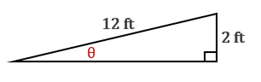Chapter 11.CT, Problem 12CTElementary Geometry For College St...

7th Edition
Alexander + 2 others
ISBN: 9781337614085

Solutions

Chapter
SectionElementary Geometry For College St...

7th Edition
Alexander + 2 others
ISBN: 9781337614085
Textbook Problem

A roofline shows a span of 12 ft across a sloped roof, and thisspan is accompanied by a 2-ft rise. To the nearest degree, findthe measure of θ.

To determine

To find:

The measure of θ to the nearest degree.

Explanation

Given:

A roofline shows a span of 12 ft across a sloped roof, and this span is accompanied by a 2-ft rise.

Approach:

In a right triangle, the sine ratio for an acute angle is the ratio oppsite sidehypotenuse.

Calculation:

From the diagram, opposite side = 2 ft

Still sussing out bartleby?

Check out a sample textbook solution.

See a sample solution

The Solution to Your Study Problems

Bartleby provides explanations to thousands of textbook problems written by our experts, many with advanced degrees!

Get Started

Find each sum 47+6.3+20.71+170.027

Elementary Technical Mathematics

Differentiate the functions in Problems 3-20. 12.

Mathematical Applications for the Management, Life, and Social Sciences

Find the distance between (2, 4) and (6, 8).

Applied Calculus for the Managerial, Life, and Social Sciences: A Brief Approach

Find the limit. limu1u41u3+5u26u

Single Variable Calculus: Early Transcendentals, Volume I

A population of N = 15 scores has SX = 120. What is the population mean?

Statistics for The Behavioral Sciences (MindTap Course List)

Convert the expressions in Exercises 6584 to power form. x23

Finite Mathematics and Applied Calculus (MindTap Course List)

Find y and y. y=eex

Single Variable Calculus: Early Transcendentals

Prove the identity cos2x=1tan2x1+tan2x.

Trigonometry (MindTap Course List)

. ∞ −∞ 1 −1

Study Guide for Stewart's Single Variable Calculus: Early Transcendentals, 8th